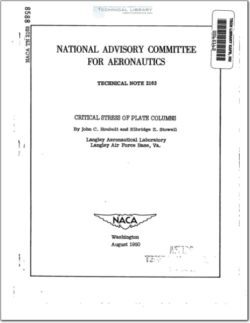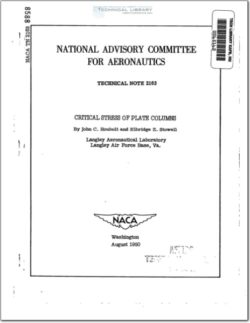17,004 Documents in our Technical Library

AA-CP-20212-001
AA-CP-20212-001
ADPO10769 Occurrence of Corrosion in Airframes
The purpose of this lecture is to provide an overview ...
MIL-STD-1759 Rivets and Rivet Type Fasteners Preferred for Design
The purpose of this book form standard is to provide ...
MIL-STD-810G Environmental Engineering Considerations and Laboratory Tests
This standard contains materiel acquisition program planning and engineering direction ...
MIL-R-7885D General Specification for Rivets, Blind, Structural, Mechanically Locked Spindle and Friction Locked Spindle
This specification covers mechanically locked spindle and  friction locked spindle ...

### naca-tn-2163• Version
• 668.97 KB File Size
• 1 File Count
• May 5, 2016 Create Date
• May 5, 2016 Last Updated
Scroll for Details

National Advisory Committee for Aeronautics, Technical Notes - Critical Stress of Plate ColumnsSolutions are given for the elastic buckling stress of flat
rectangular plates with simply supported or fixed ends when loaded
as columns. for the case of simply supported ends, an exact solution
is made; for the case of fixed ends, an approximte solution is made
by use of a power series in conjunction with Lagrangian undetermined
multipliers. The critical stress is given in terms of the Euler
value of the load multiplied by a coefficient which depends on the
width-length ratio of the column and varies for practical purposes
between the values 1 and 1 , where u is Poisson's ratio of the
material.
The results showed that the plates may be considered as "colmns,"
as the term is used in ordinary engineering practice, when the width-
length ratio is less than 0.1 and may be considered as infinitely wide
plates (Euler column value times coefficient of i—é when the
width-length ratio is greater than 10. For intermediate width-length
ratios, the appropriate coefficient my be found from a chart.
The procedure for determining the critical stress of plate columns
with intermediate end fixities is also given. The study also indicates
the solution for the local buckling of angle or cruciform sections.
The Euler equation for column buckling is recognized to be correct
for a very narrow rectangular plate loaded as a column (reference 1)
and is generally assumed to be modified by a factor when
applied to a very wide plate. This factor is introduced on the basis
that the end supports force the plate to buckle into a cylindrical
surface over most of its width, and thereby ,the bending stiffness is
increased from E1 to I7. (See reference 2.) For the very
narrow plate, the effect of the end supports is negligible because
only a small region at each end is affected (St. Venant's principle).
Thus, the critical stress may change as much as 10 percent from the
Euler value, depending on whether the' plate column is very narrow or
very wide.

FileAction
naca-tn-2163 Critical Stress of Plate Columns.pdf

### naca-tn-2163• Version
• 668.97 KB File Size
• 1 File Count
• May 5, 2016 Create Date
• May 5, 2016 Last Updated
Scroll for Details

National Advisory Committee for Aeronautics, Technical Notes - Critical Stress of Plate ColumnsSolutions are given for the elastic buckling stress of flat
rectangular plates with simply supported or fixed ends when loaded
as columns. for the case of simply supported ends, an exact solution
is made; for the case of fixed ends, an approximte solution is made
by use of a power series in conjunction with Lagrangian undetermined
multipliers. The critical stress is given in terms of the Euler
value of the load multiplied by a coefficient which depends on the
width-length ratio of the column and varies for practical purposes
between the values 1 and 1 , where u is Poisson's ratio of the
material.
The results showed that the plates may be considered as "colmns,"
as the term is used in ordinary engineering practice, when the width-
length ratio is less than 0.1 and may be considered as infinitely wide
plates (Euler column value times coefficient of i—é when the
width-length ratio is greater than 10. For intermediate width-length
ratios, the appropriate coefficient my be found from a chart.
The procedure for determining the critical stress of plate columns
with intermediate end fixities is also given. The study also indicates
the solution for the local buckling of angle or cruciform sections.
The Euler equation for column buckling is recognized to be correct
for a very narrow rectangular plate loaded as a column (reference 1)
and is generally assumed to be modified by a factor when
applied to a very wide plate. This factor is introduced on the basis
that the end supports force the plate to buckle into a cylindrical
surface over most of its width, and thereby ,the bending stiffness is
increased from E1 to I7. (See reference 2.) For the very
narrow plate, the effect of the end supports is negligible because
only a small region at each end is affected (St. Venant's principle).
Thus, the critical stress may change as much as 10 percent from the
Euler value, depending on whether the' plate column is very narrow or
very wide.

FileAction
naca-tn-2163 Critical Stress of Plate Columns.pdf
17,004 Documents in our Technical Library